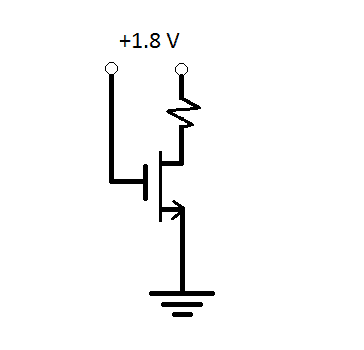# Transistor analysis

## Homework Statement

Just started learning about transistors, and I am having difficulty doing this problem.The transistor in the circuit has $$k_n ^'=0.5mA/V^2, V_t=0.5 V, \lambda =0.$$
Show that operation at the edge of saturation is obtained when the following condition is satisfied
$$(\frac{W}{L})R_D=1.5 kΩ$$

## The Attempt at a Solution

As far as I know, if it is operating in on the edge of saturation, then
$$\frac{1}{2}k_m ^'(\frac{W}{L})v_{OV}$$
However, when I manipulate this equation, I am unable to get to $$(\frac{W}{L})R_D=1.5 kΩ$$

Can anyone provide me some guidance on this?